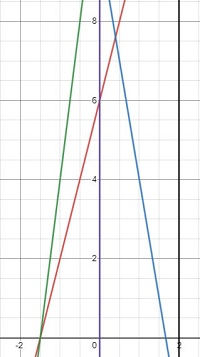# Program to find how many lines intersect in Python

Suppose we are given a list that contains values in pairs of (m, c). These values represent a line, where y = mx + c. We are also given two values, l, and r. We have to find out the number of the lines that intersect with each other between the range x = l to x = h.

So, if the input is like input_list = [[4, 6],[-6, 10],[8, 12]], l = 0, h = 2, then the output will be 2.If we look at the given photo, the lines 4x + 6 = 0 and -6x + 10 intersect within the given range. So, there are two lines that are intersecting, so the output is 2.

To solve this, we will follow these steps −

• seg := a list containing pairs [(m * l + c, m * h + c, i) for index i, and values (m, c) in input_list]
• sort the list seg
• ans := a new list of the size of input_list containing 0s
• c := a new map from seg
• for each (x, y, i) in seg, do
• if c[x] > 1, then
• ans[i] := 1
• max_c := -(10 ^ 10)
• prv := -(10 ^ 10)
• for each (x, y, i) in seg, do
• if x is same as prv, then
• ans[i] := 1
• if y <= max_c, then
• ans[i] := 1
• max_c := maximum of (max_c, y)
• prv := x
• min_c = 10 ^ 10
• prv = 10 ^ 10
• for each (x, y, i) in seg reversed, do
• if x is same as prv, then
• ans[i] := 1
• if y >= min_c, then
• ans[i] := 1
• min_c := minimum of (min_c, y)
• prv := x
• return sum of the elements of list (ans)

## Example

Let us see the following implementation to get better understanding −

from collections import Counter

def solve(input_list, l, h):
seg = [(m * l + c, m * h + c, i) for i, (m, c) in
enumerate(input_list)]
seg.sort()
ans = [0 for _ in input_list]
c = Counter(seg)
for (x, y, i) in seg:
if c[x] > 1:
ans[i] = 1
max_c = -(10 ** 10)
prv = -(10 ** 10)
for (x, y, i) in seg:
if x == prv:
ans[i] = 1
if y <= max_c:
ans[i] = 1
max_c = max(max_c, y)
prv = x
min_c = 10 ** 10
prv = 10 ** 10
for (x, y, i) in seg[::-1]:
if x == prv:
ans[i] = 1
if y >= min_c:
ans[i] = 1
min_c = min(min_c, y)
prv = x
return sum(ans)

print(solve([[4, 6],[-6, 10],[8, 12]], 0, 2))

## Input

[[4, 6],[-6, 10],[8, 12]], 0, 2

## Output

2